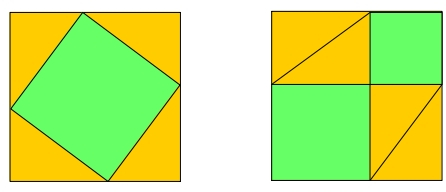# Welcome

### This site reflects my interests in computer programming and mathematics.The two large squares are the same size. All the orange triangles are the same size. So, if you remove the four triangles from the left square, and remove the four triangles from the right square, the area remaining on the left and the area remaining on the right are equal. But the green square on the left is the square of the hypotenuse of the triangle, and the green squares on the right are the squares of the two legs of the triangle. Thus, we have a visual proof of Pythagoras' Theorem: The sum of the squares of the legs of a right triangle is equal to the square of the hypotenuse.

There is an old story (possibly true, maybe not) that the image above was found chiseled on a large rock in India in ancient times, with one word underneath: BEHOLD!